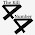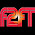## Tuesday, December 30, 2014

### 33 | Terabyte

Terabyte = 2+5+9+1+2+7+2+5 = 33
Terabyte = 20+5+18+1+2+25+20+5 = 96

#### 4 comments:

1.Tera can mean trillion.

As we move to the era of the Trillionaire, with the 44th President, 44 Democrats in the Senate, along with the 114th U.S. Congress. With Senate Pres. pro tem Orrin Hatch and House Speaker John Boehner both having names that sum to 114, and all the other curiosities - My thought is: Beware of the Tera Era - its gonna scare ya.

Tera = 20+5+18+1 = 44

Orrin Hatch = 15+18+18+9+14+8+1+20+3+8 =114
John Boehner = 10+15+8+14+2+15+5+8+14+5+18 = 114

2.Yes, I have posts on Orrin and John. Don't forget we voted on the 114th Congress on 11/4.
Orrin = 15+18+18+9+14 = 74... thus the 'double R'

3.Also, I really like that phrase, beware the tera era...' I can see Al Pacino's character saying it in Scent of a Woman.

4.11/4, wow thats right. So strange.